# Class 9 NCERT Solutions- Chapter 12 Heron’s Formula – Exercise 12.2

• Difficulty Level : Medium
• Last Updated : 04 Mar, 2021

### Question 1. A park, in the shape of a quadrilateral ABCD, has ∠ C = 90º, AB = 9 m, BC = 12 m, CD = 5 m and AD = 8 m. How much area does it occupy?

Solution:

Given, a quadrilateral ABCD where ∠C = 90º.

Attention reader! All those who say programming isn't for kids, just haven't met the right mentors yet. Join the  Demo Class for First Step to Coding Coursespecifically designed for students of class 8 to 12.

The students will get to learn more about the world of programming in these free classes which will definitely help them in making a wise career choice in the future.

Construction: Join diagonal BD.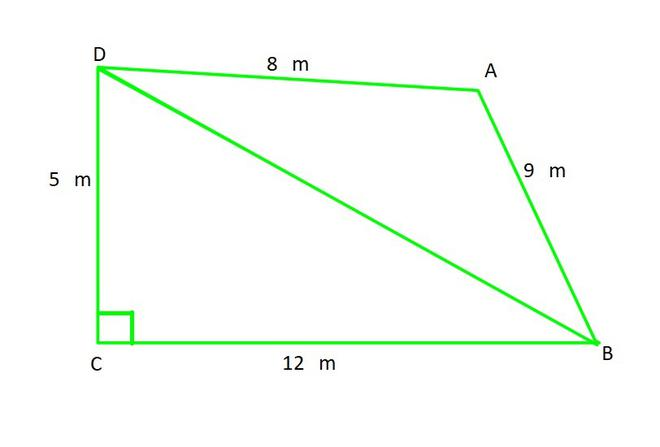As we can see that, △DCB is right-angled at C

Hence, BC is the base and CD is height of △DCB, so

ar(△DCB) =× Base × Height× 12 × 5

= 30 m2……………………………………(1)

As △DCB is right angle triangle we can calculate third side by Pythagoras theorem

BD2 = CB2 + CD2

BD2 = 122 + 52

BD = √(144+25)

BD = √169

BD =13 m

Now, Area of △DAB can be calculated by Heron’s Formula, where

AB = a = 9 m

AD = b = 8 m

BD = c = 13 m

Semi Perimeter (s) =s =s = 15 m

ar(△DAB) = √s(s-a)(s-b)(s-c)

= √15(15-9)(15-8)(15-13)

= √15×(6)×(7)×(2)

= 6√35 m2……………………………………..(2)

From (1) and (2), we can conclude that,

ar(ABCD) = ar(△DCB)+ar(△DAB)

= (30 + 6√35)

= (30 + 35.5)

≈ 65.5 m2 (approx.)

### Question 2. Find the area of a quadrilateral ABCD in which AB = 3 cm, BC = 4 cm, CD = 4 cm, DA = 5 cm and AC = 5 cm.

Solution: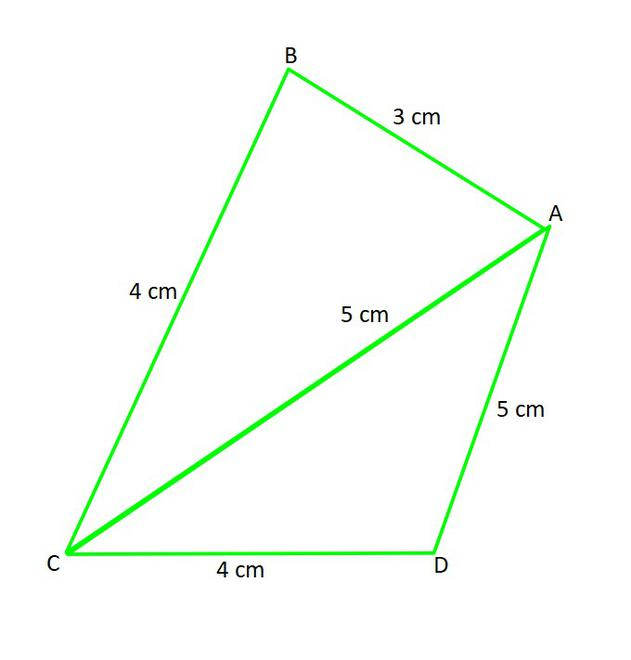Here, we can notice that in △ABC

AC2 = AB2 + BC2

52 = 32 + 42

25 = 25

LHS = RHS

As this triangle is satisfying the Pythagoras theorem, Therefore, △ABC is a right angle triangle, 90° at B.

Hence, BC is the base and AB is height of △ABC. so

So, ar(△ABC) =× Base × Height× 4 × 3

= 6 cm2……………………………….(1)

Now, Area of △DAC can be calculated by Heron’s Formula, where

AD = a = 5 cm

DC = b = 4 cm

AC = c = 5 cm

Semi Perimeter (s) =s =s = 7 cm

ar(△DAC) = √s(s-a)(s-b)(s-c)

= √7(7-5)(7-4)(7-5)

= √7×(2)×(3)×(2)

= 2√21 cm2……………………………………..(2)

From (1) and (2), we can conclude that,

ar(ABCD) = ar(△ABC)+ar(△DAC)

= (6 + 2√21)

= (6 + 9.2(approx.))

≈ 15.2 cm2 (approx.)

### Question 3. Radha made a picture of an aeroplane with coloured paper as shown in (Fig). Find the total area of the paper used.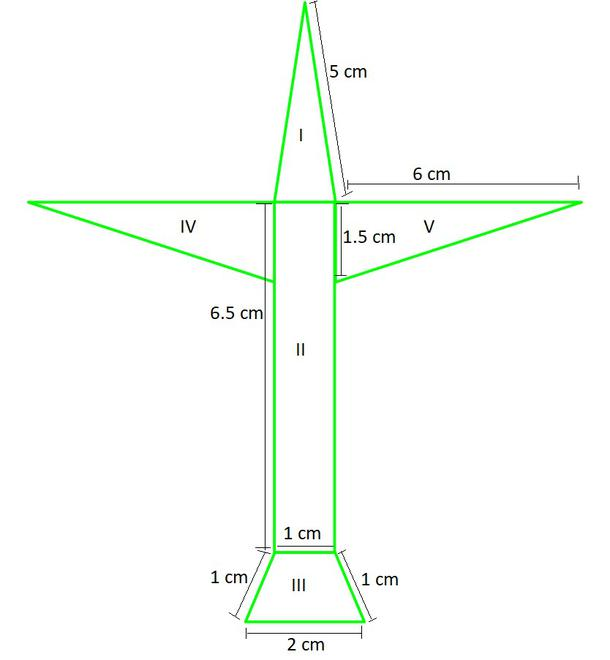Solution:

Total area of the paper used = (Area I + Area II + Area III + Area IV + Area V)

Area I: Triangle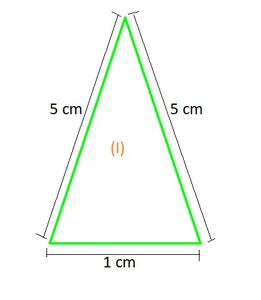Now, Area of triangle can be calculated by Heron’s Formula, where

a = 5 cm

b = 5 cm

c = 1 cm

Semi Perimeter (s) =s =s = 5.5 cm

ar(△) = √s(s-a)(s-b)(s-c)

= √5.5(5.5-5)(5.5-5)(5.5-1)

= √5.5×(0.5)×(0.5)×(4.5)

= 0.5×0.5×3√11

= 0.75√11

≈ 2.5 cm2 ……………………………………..(1)

Area II: Rectangle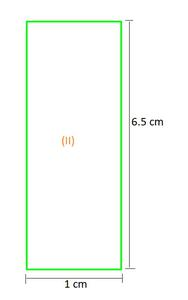= 1 × 6.5 = 6.5 cm2……………………………….(2)

Area III: Trapezium = Area of parallelogram EFAO + △ AFD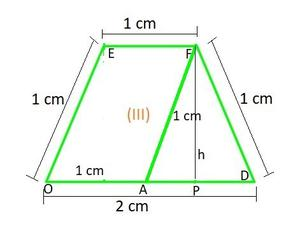OD = 2cm

AD = OD-OA = 2-1 = 1 cm

Hence, △ AFD is equilateral.

PD =AD =cm

△ PFD is right angled at P, Pythagoras theorem is applicable

12=h2 +()2

h = √(1-)

h = √cm

Area III:

= (Base × Height) + (× Base × Height)

= (1 ×) + (× 1 ×)= 1.29 cm2…………………………………(3)

Area IV and V: 2 times Triangle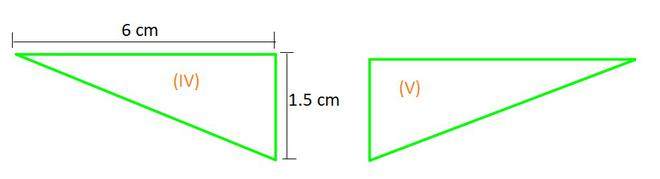ar(△) =× Base × Height× 6 × 1.5

= 4.5 cm2

Area IV + Area V = 2×ar(△)

= 2×4.5

= 9 cm2 …………………………………(4)

Hence, Total area of the paper used = (Area I + Area II + Area III + Area IV + Area V)

= (1) + (2) + (3) + (4)

= 2.5 + 6.5 + 1.29 +9

= 19.29 cm2

### Question 4. A triangle and a parallelogram have the same base and the same area. If the sides of the triangle are 26 cm, 28 cm and 30 cm, and the parallelogram stands on the base 28 cm, find the height of the parallelogram.

Solution: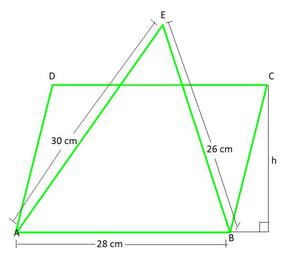Now, Area of △AEB can be calculated by Heron’s Formula, where

AE = a = 28 cm

EB = b = 30 cm

AB = c = 26 cm

Semi Perimeter (s) =s = (28+30+26)/2

s = 42 cm

ar(△AEB) = √s(s-a)(s-b)(s-c)

= √42(42-28)(42-30)(42-26)

= √42×(14)×(12)×(16)

= 336 cm2

As it is given, ar(△AEB) = ar(parallelogram ABCD)

336 = Base × Height

336 = 28 × h

h =h = 12 cm

Hence, the height of the parallelogram = 12 cm

### Question 5. A rhombus shaped field has green grass for 18 cows to graze. If each side of the rhombus is 30 m and its longer diagonal is 48 m, how much area of grass field will each cow be getting?

Solution:

ABCD is a rhombus having diagonal AC = 48 cm

Diagonal of Rhombus divides the area into two equal parts.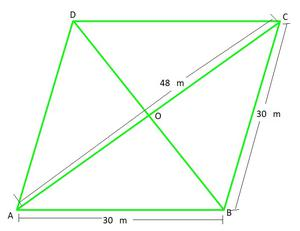Now, ar(△ABC) can be calculated by Heron’s Formula, where

AB = a = 30 m

BC = b = 30 m

AC = c = 48 m

Semi Perimeter (s) =s =s = 54 m

ar(△ABC) = √s(s-a)(s-b)(s-c)

= √54(54-30)(54-30)(54-48)

= √54×(24)×(24)×(6)

= 432 m2

Hence, Area of rhombus = 2 × (ar(△))

= 2 × 432 = 864 m2

Area for 18 cows = 864 m2

Area for each cow = 864 / 18 = 48 m2

### Question 6. An umbrella is made by stitching 10 triangular pieces of cloth of two different colours (see Fig. 12.16), each piece measuring 20 cm, 50 cm and 50 cm. How much cloth of each colour is required for the umbrella?

Solution:

Let’s consider for each triangle.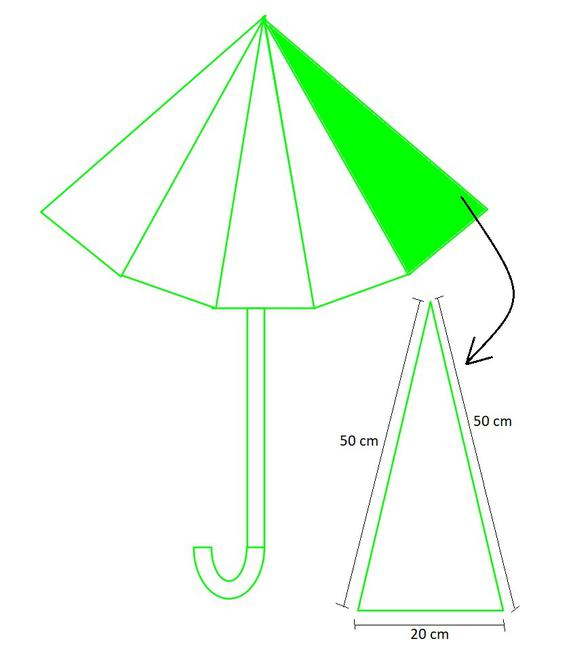Now, for each ar(△)can be calculated by Heron’s Formula, where

a = 50 cm

b = 50 cm

c = 20 cm

Semi Perimeter (s) =s =s = 60 cm

ar(△) = √s(s-a)(s-b)(s-c)

= √60(60-50)(60-50)(60-20)

= √60×(10)×(10)×(40)

= 200√6 cm2

Hence, the Total Area = 5×200√6

= 1000√6 cm2

### Question 7. A kite in the shape of a square with a diagonal 32 cm and an isosceles triangle of base 8 cm and sides 6 cm each is to be made of three different shades as shown in Fig. How much paper of each shade has been used in it?

Solution: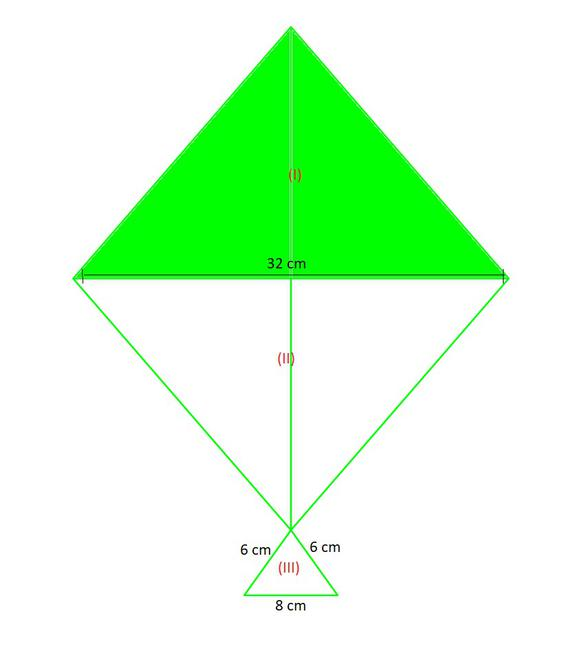As the area of kite is in the square, it area will be

Area of kite =×(diagonal)2×32×32

= 512 cm2

Diagonal divides area into equal areas.

Area of kite = Area I + Area II

512 = 2 × Area I

Area I =Area II = 256 cm2……………………………..(1)

Area III: Area of triangle

Now, for each ar(△)can be calculated by Heron’s Formula, where

a = 6 cm

b = 6 cm

c = 8 cm

Semi Perimeter (s) =s =s = 10 cm

ar(△) = √s(s-a)(s-b)(s-c)

= √10(10-6)(10-6)(10-8)

= √10×(4)×(4)×(2)

= 8√5 cm2………………………………(2)

### Question 8. A floral design on a floor is made up of 16 tiles which are triangular, the sides of the triangle being 9 cm, 28 cm and 35 cm (see Fig). Find the cost of polishing the tiles at the rate of 50p per cm2.

Solution: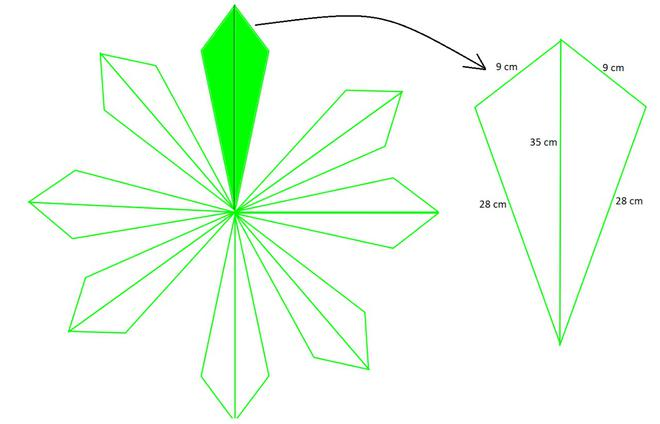Area for each triangle will be:

Now, for each ar(△)can be calculated by Heron’s Formula, where

a = 9 cm

b = 28 cm

c = 35 cm

Semi Perimeter (s) =s =s = 36 cm

ar(△) = √s(s-a)(s-b)(s-c)

= √36(36-9)(36-28)(36-35)

= √36×(27)×(8)×(1)

= 36√6 cm2

As there are 16 tiles, so total area = 16 × 36√6

= 1410.906 cm2

As 1 cm2 = 50 p = ₹ 0.5

1410.906 cm2 = ₹ 0.5 ×1410.906

= ₹ 705.45

### Question 9. A field is in the shape of a trapezium whose parallel sides are 25 m and 10 m. The non-parallel sides are 14 m and 13 m. Find the area of the field.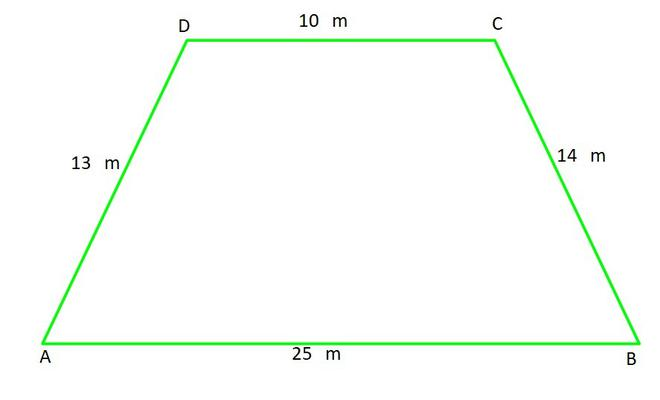Solution: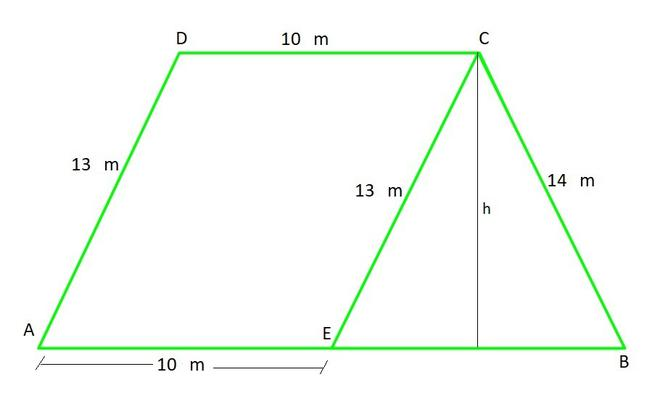AB = 25 m

EB = AB-AE = 25-10 = 15 m

Now, for ar(△ECB) can be calculated by Heron’s Formula, where

a = 13 m

b = 14 m

c = 15 m

Semi Perimeter (s) =s =s = 21 m

ar(△ECB) = √s(s-a)(s-b)(s-c)

= √21(21-3)(21-14)(21-15)

= √21×(18)×(7)×(6)

= 84 m2 ………………………….(1)

ar(△ECB) =× Base × Height

84 m2× 15 × h

h =m

h = 11.2 m

Total Area = Area of parallelogram AECD + ar(△ECB)

= (Base × Height) + 84m

= 10 × 11.2 + 84

Total Area = 196 m2

My Personal Notes arrow_drop_up